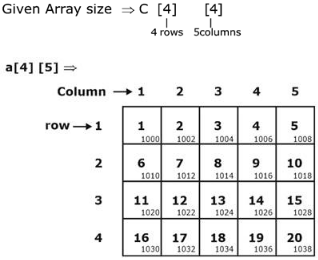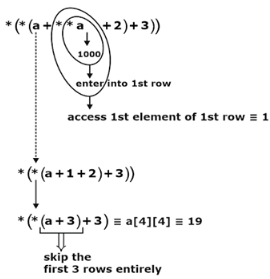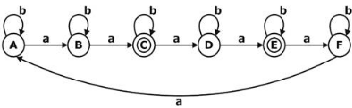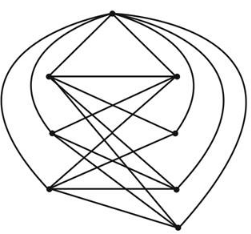# GATE Solved Paper 2020 - GATE 2020

Int main() {
Int a={{1, 2, 3, 4, 5},
{6, 7, 8, 9, 10},
{11, 12, 13, 14, 15},
{16, 17, 18, 9, 20}};
Printf(“%d\n”, *(*(a+**a+2)+3);
Return(0);
}
The output program is ______ .

Note – Numerical Type question

• A

19• B

20• C

18• D

20• Option : A
• Explanation :base address of a = 1000
“*” → has higher precedence the “+”
*(*(a + ** a + 2) + 3)• A

5• B

4• C

6• D

3• Option : C
• Explanation :
L = w ϵ (a, b)* |na(w) = multiple of 2 but not 3.
L1 = {na mod 2 = 0} ⇒ need 2 states
L2 = {na mod 3 ≠ 0} ⇒ need 3 states
|L1 × L2| = (2 × 3) = 6 states.
Minimal DFA design ⇒∴ No. of states in min. DFA = 6 states.

• A

14• B

13• C

12• D

11• Option : A
• Explanation :⇒ # of instruction possible = 24 = 16
∴ No. of free opcodes = 16 – x
Let x is number of R-type instruction exist
∴ No. of I-type instruction possible = (16 – x) * 22
8 = 64 – 4x
4x = 64 – 8 = 56
x = 14

• A

11• B

10• C

13• D

12• Option : D
• Explanation :
LILAC
IAC
3! + LLAC
3 + 4/2 + 3 + 4/2 + 4/2
3 + 2 + 3 + 2 + 2 = 12

• A

7• B

6• C

5• D

8• Option : A
• Explanation :Minimum number = 3 + 4
Of edge-colors required = 7
Related Quiz.
GATE 2020